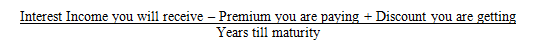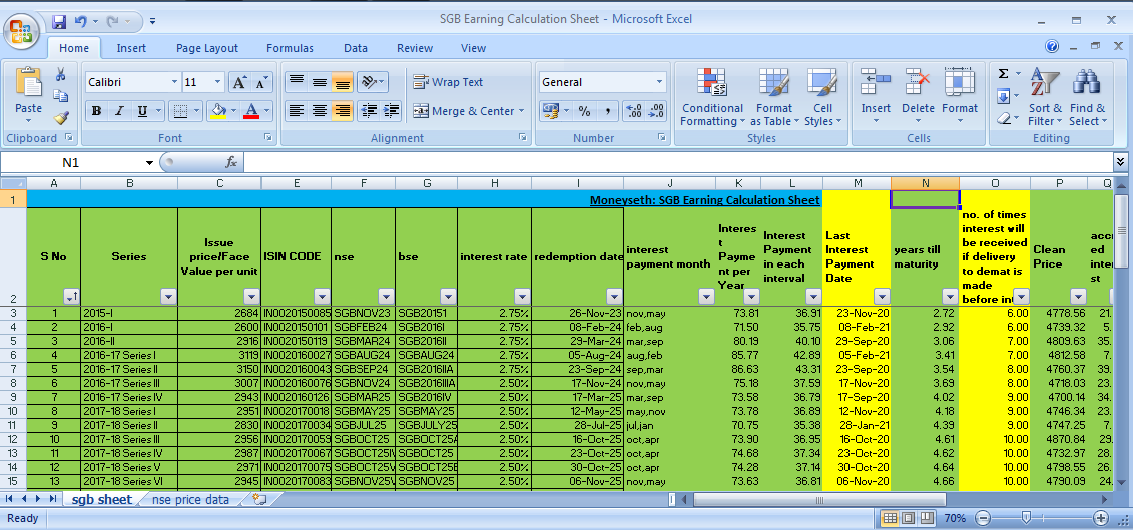# Sovereign Gold Bond Calculator

##### Here in this post you will get to know about how to calculate sovereign gold bond return easilyMarch 7, 2021, 12:23 p.m.

Sovereign Gold BondAs per the Reserve Bank of India SGBs are government securities denominated in grams of gold. These are the substitutes for holding physical gold. Investors have to pay the issue price in cash and the bonds will be redeemed to them in cash on maturity. The Bond is issued by the Reserve Bank of India on behalf of the Government of India. The issue price and redemption price depends on the price of gold as per the IBJA Rate. Here, we also get a return in form of Interest Income for every Sovereign Gold Bond investment, which is determined at the time of issue. The Interest rate is calculated on the face value amount and generally, it is 2.5% per annum. Here, we receive interest every 6 months till maturity or redemption date.

There are many Sovereign Gold Bonds issued by RBI, first issue was made on 26th November 2015, where the interest rate was provided at 2.75% on Face value. At that time the issue price was Rs. 2684 per unit. Till now there are over 40 issues and the latest issue got opened from 1st March 2021 to 5th March 2021. Here the issue price or face value was Rs. 4662 and the coupon rate(Interest Rate) was determined as 2.5% on face value.

Now let’s talk about Sovereign Gold Bond Calculator. First, you have to know that every sovereign gold bonds are listed on stock exchanges and for each, we get a different return so first, we have to get the data about each sovereign gold bond because each series contains a different face value and different coupon rate (How to Buy Sovereign Gold Bonds from Secondary Market). Due to these differences, each sovereign gold bond provides a different return (click here to get all sovereign gold bond data). For example, let’s assume that you are buying SGBAUG28V on 11th February 2021 which has a face value amount of Rs. 5333/- and coupon rate 2.5%, here the interest income is Rs. 133.32 per annum and we get interest payout 2 times in a year at Rs. 66.66 and the redemption date is 11th August 2028. Also, you have to get the real-time price of Gold (999Purity) as per IBJA Rates (let’s assume Rs. 4600) and the price you have to pay (let’s assume Rs. 4700). After getting all the relevant data you can now calculate the earnings of the sovereign gold bond by using this simple formulaIn this example of SGBAUG28V, we will generate an Annualized Return of 2.58% as per the formula given above.

Here to calculate your premium or discount you have to subtract the buying price of SGB from the price of gold as per IBJA Rates. If you are getting a positive figure then it is a discount and if you are getting a negative figure then you are paying a premium for buying that SGB.

And to calculate years till maturity you just need your buying date and the redemption date of SGB. After subtracting the buying date from the SGB redemption date you will get the years till maturity figure.

To calculate interest income you just need to multiply interest income for every 6 months and the number of times you are going to receive the interest payout till maturity.

We can easily calculate the sovereign gold bond return by using an excel sheet if you need the SGB Calculation Excel Sheet then you can contact me on 7003419137. or you can watch this video for reference https://youtu.be/uwYvyBYpgjc for more details on gold bonds and other money related details you can follow my youtube channel Sunny SethTags - Sovereign Gold Bond Gold SGB Sovereign Gold Bond Return Calculator